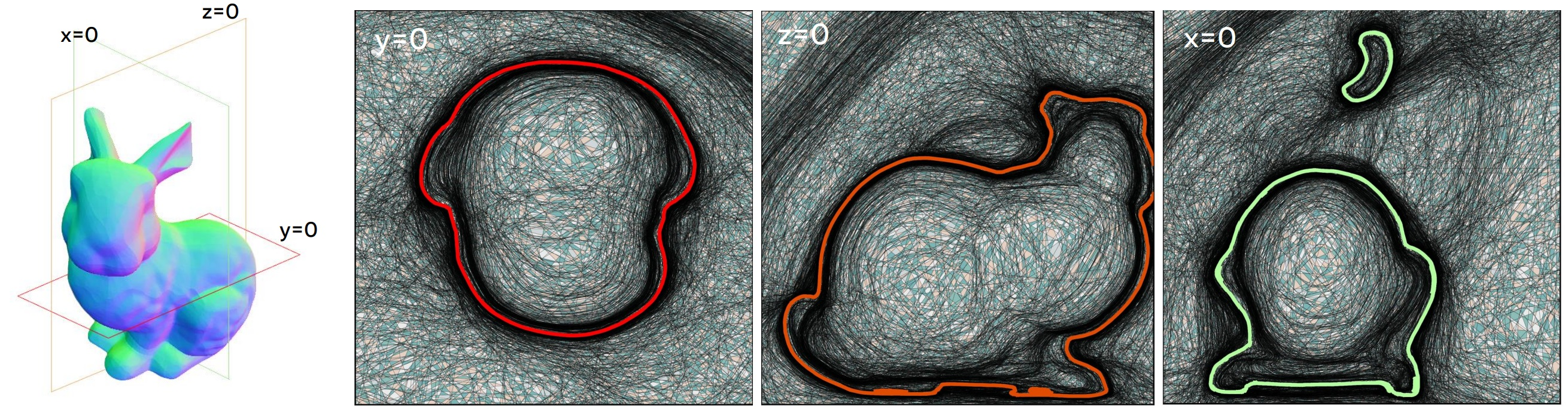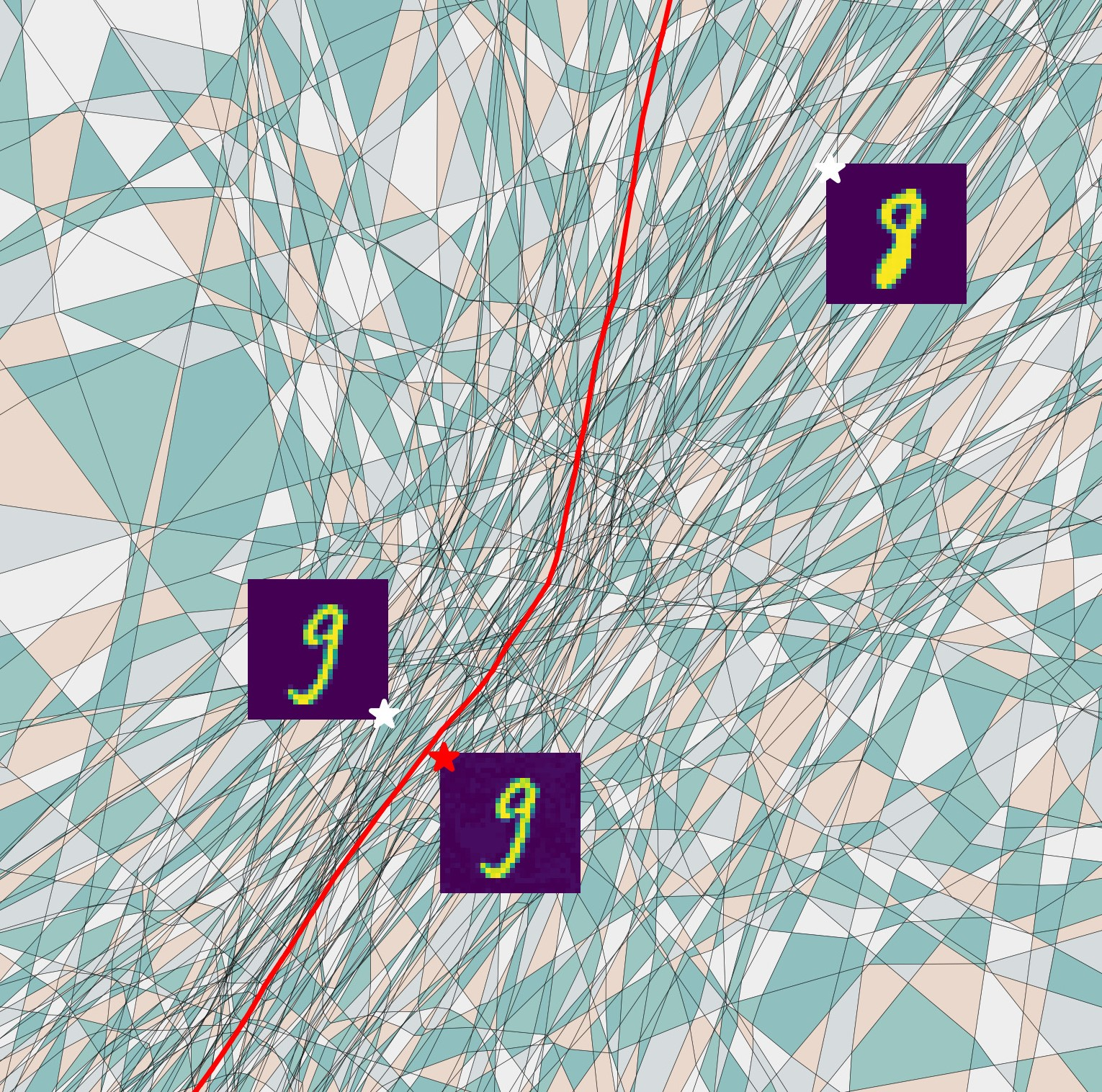## SplineCam: Exact Visualization and Characterization of Deep Network Geometry and Decision Boundary CVPR 2023 Highlight

Video: Evolution of the partition formed by a depth 5 and width 10 binary classifier MLP, while training on two circles (left) and two moons (right). Dark red line denotes the learned decision boundary, and black lines denote the region boundaries. Norm of the region-wise slope parameters is used to color each region.

### AbstractCurrent Deep Network (DN) visualization and interpretability methods rely heavily on data space visualizations such as scoring which dimensions of the data are responsible for their associated prediction or generating new data features or samples that best match a given DN unit or representation. In this paper, we go one step further by developing the first provably exact method for computing the geometry of a DN’s mapping – including its decision boundary – over a specified region of the data space. By leveraging the theory of Continuous Piece-Wise Linear (CPWL) spline DNs, SplineCam exactly computes a DN’s geometry without resorting to approximations such as sampling or architecture simplification. SplineCam applies to any DN architecture based on CPWL nonlinearities, including (leaky-)ReLU, absolute value, maxout, and max-pooling and can also be applied to regression DNs such as implicit neural representations. Beyond decision boundary visualization and characterization, SplineCam enables one to compare architectures, measure generalizability and sample from the decision boundary on or off the manifold.

### Exact Visualization of DNN partition geometry

Video: Exact computation allows visualizing partitions upto machine precision. Here we are zooming into the partition of a randomly initialized MLP.Video: (Left) SplineCam can be used to monitor changes in the learned function during training. Here regions are colored by the norm of their slope. (Right) Visualize and compute the margin of adversarial examples from the decision boundary. Here the red star corresponds to an adv. example generated via PGD. Regions colored randomly.

### Monitor Evolution of Decision Boundary During TrainingDecision boundary of a 5 layer convnet during training epochs {50,100,150,200,300} (columns) trained on a binary classification task to classify between Egyptian cat and Tabby Cat classes of tinyimagenet. White points are images from the dataset that were used as anchor points for the 2d-plane cut in the input space. First, we clearly observe that in the first two columns (epoch 50 and 100) not much change occurs to the decision boundar (red line). However, between epoch 100 and 150, a sudden change occurs making the decision boundary move out of the 2D-slice, which then slowly recovers until the end of training (top row). On the other hand when looking at a different 2D-slide of the input space (bottom row) we can see that again between epoch 50 and 100 training does not drastically update the decision boundary, but that between epoch 100 and 150, a sudden change in the decision boundary occurs. It then stays roughly identical (within this slice) until the end of training.

### Fast Computation of Partition GeometryGiven an input domain as a polygonal region and a set of hyperplanes, SplineCam first produces a graph using all the edgehyperplane and hyperplane-hyperplane intersections. To find all the convex cycles in the graph, we select a boundary edge (blue arrow), do a breadth first search to find the shortest path through the graph between the two nodes and obtain the adjacent region (blue). While performing the traversal we enqueue the traversed edges for repetition. For each of the enqueued edges, we repeat the process to obtain the neighboring regions. Each non-boundary edge is allowed to be traversed twice, once from either direction. Once regions are found, we obtain a new set of hyperplanes corresponding to deeper layers and create partition graphs for each region.Growth of the number of regions with width (Left) and runtime of our algorithm (right) for a single layer randomly initialized ReLU neural network with variable width (n). Solid lines represent different input space dimensionality. For all the input dimensions, we take a randomly oriented square 2D domain centered on the origin and compute the input space partitioning on this domain. With increased input dimensionality, we see a slight reduction in number of regions and runtime.

### Characterize Input Space Regions via Partition StatisticsAverage partition statistics for 90 tinyimagenet test samples with and without DA training for VGG11 and VGG16. The average volume and number of regions are indicative of partition density whereas eccentricity is indicative of the shape of the regions. For VGG11 a distinct difference in the statistics can be visualized between DA and non-DA training. DA training significantly increases the partition density at test points, which is indicative of better generalizability. On the other hand, the difference reduces for VGG16 while the overall region density increases. This is expected behavior since the VGG16 has significantly more parameters. For both case, the DA models acquired a comparable accuracy on the tinyimagenet-200 classification task